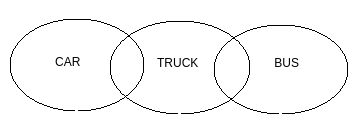Q

# Directions: Given two statements, verify the conclusions and mark the answer as given below.

Directions : Given two statements, verify the conclusions and mark the answer as given below.

Statements:

I.          Some cars are trucks                 II.          Some trucks are buses

Conclusions:

I,.         Some cars are trucks                 II.          Some cars are not trucks

• Option 1)

if only conclusion I follow.

• Option 2)

If only conclusion II follows.

• Option 3)

If either conclusion I or II follows.

• Option 4)

If neither of the two conclusions follows.

• Option 5)

If both conclusions follow

ViewsI. Follows

II. Not Follows

Exams
Articles
Questions